# NY Regents - Triangles and Congruency: Help and Review Chapter Exam

Exam Instructions:

Choose your answers to the questions and click 'Next' to see the next set of questions. You can skip questions if you would like and come back to them later with the yellow "Go To First Skipped Question" button. When you have completed the practice exam, a green submit button will appear. Click it to see your results. Good luck!

### Page 1

#### Question 1 1. In the pictured triangle, which of the following lines is an altitude?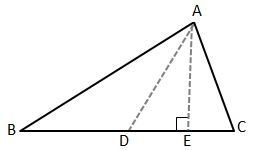#### Question 3 3. What additional information do you need to prove that triangle ABC is congruent to triangle DEF using the HA theorem?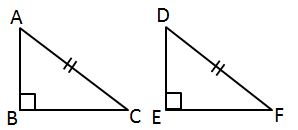#### Question 4 4. What type of line segment is represented by the dotted line in the pictured triangle?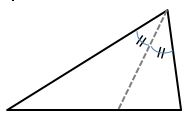### Page 2

#### Question 6 6. What type of triangle is pictured?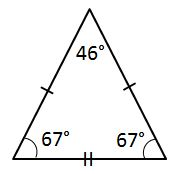#### Question 7 7. Which theorem can be used to establish congruence with the pictured triangles?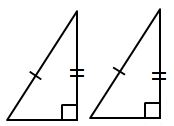#### Question 8 8. AD is a perpendicular bisector. Find the measure of AC: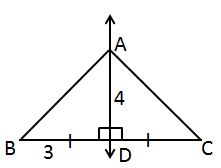#### Question 9 9. What type of line segment is represented by the vertical dotted line in the pictured triangle?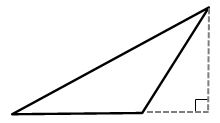### Page 3

#### Question 11 11. What is the area of the pictured rectangle?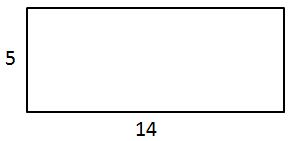#### Question 12 12. What type of triangle is pictured?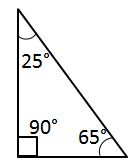#### Question 13 13. What additional information do you need to be given to prove that triangle PQS is congruent to triangle SRP using the HA theorem?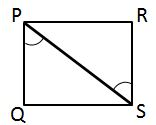#### Question 14 14. Study the given diagram. Find the value of x, the distance between T and E.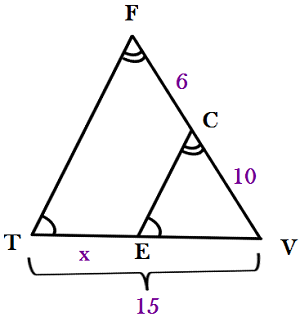#### Question 15 15. In the figure below, Angle 7 = 15x and Angle 10 = 9x + 30. Determine the measure of Angle 10.### Page 4

#### Question 16 16. In the pictured triangle, what is the centroid?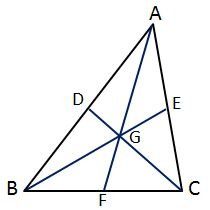#### Question 17 17. Which theorem can be used to establish congruence with the pictured triangles?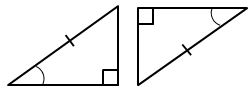#### Question 18 18. In the pictured triangle, identify the altitude(s).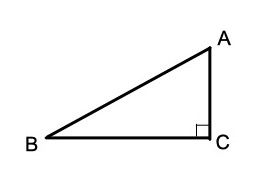#### Question 19 19. In the pictured triangle, which of these is a median?#### Question 20 20. What is the area of ABCDE, as shown in the diagram?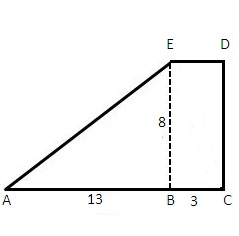### Page 5

#### Question 21 21. What type of triangle is pictured?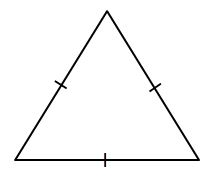#### Question 22 22. Which theorem or postulate can be used to establish congruence with the pictured triangles?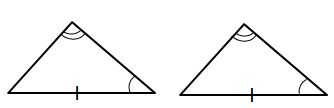#### Question 23 23. Which theorem can be used to establish congruence with the pictured triangles?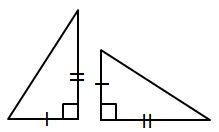#### Question 24 24. How can we explain that the pictured triangles are congruent?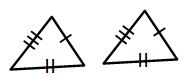#### Question 25 25. In triangle GBN, Angle G is seven more than three times a number, Angle B is five more than four times the number, and Angle N is twelve less than five times the number. Solve for the measure of Angle G.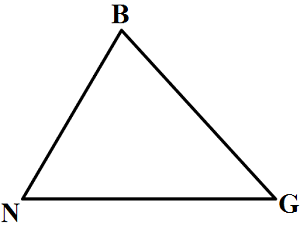### Page 6

#### Question 27 27. In the pictured triangles, what reason can we use to explain that angle QPR is congruent to angle SPT?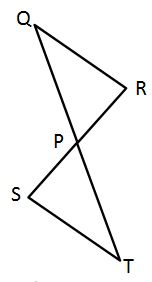#### Question 28 28. In the pictured triangle, FE is an angle bisector. What is the length of GE?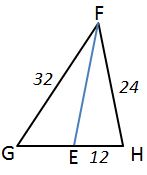#### Question 29 29. Triangle HYV and triangle AYB are similar by the AA similarity theorem. What is the value of x?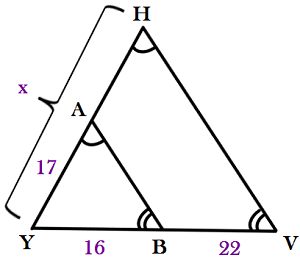#### Question 30 30. What additional information do you need to prove that triangle ABC is congruent to triangle DEF using the HL theorem?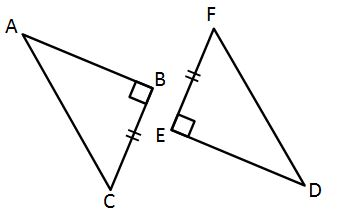#### NY Regents - Triangles and Congruency: Help and Review Chapter Exam Instructions

Choose your answers to the questions and click 'Next' to see the next set of questions. You can skip questions if you would like and come back to them later with the yellow "Go To First Skipped Question" button. When you have completed the practice exam, a green submit button will appear. Click it to see your results. Good luck!

Support